# Selina Solutions Concise Maths Class 7 Chapter 2: Rational Numbers Exercise 2E

Selina Solutions Concise Maths Class 7 Chapter 2 Rational Numbers Exercise 2E has long answer problems, according to the current syllabus of the prescribed board. Students should solve all the problems present in the textbook to understand the concepts effortlessly. It gives confidence to the students in solving the tricky problems that would appear in the annual exam. The solutions PDF is available with a free download option, so that the students can access them free of cost. For a better academic performance, Selina Solutions Concise Maths Class 7 Chapter 2 Rational Numbers Exercise 2E, PDF is available below.

## Selina Solutions Concise Maths Class 7 Chapter 2: Rational Numbers Exercise 2E Download PDF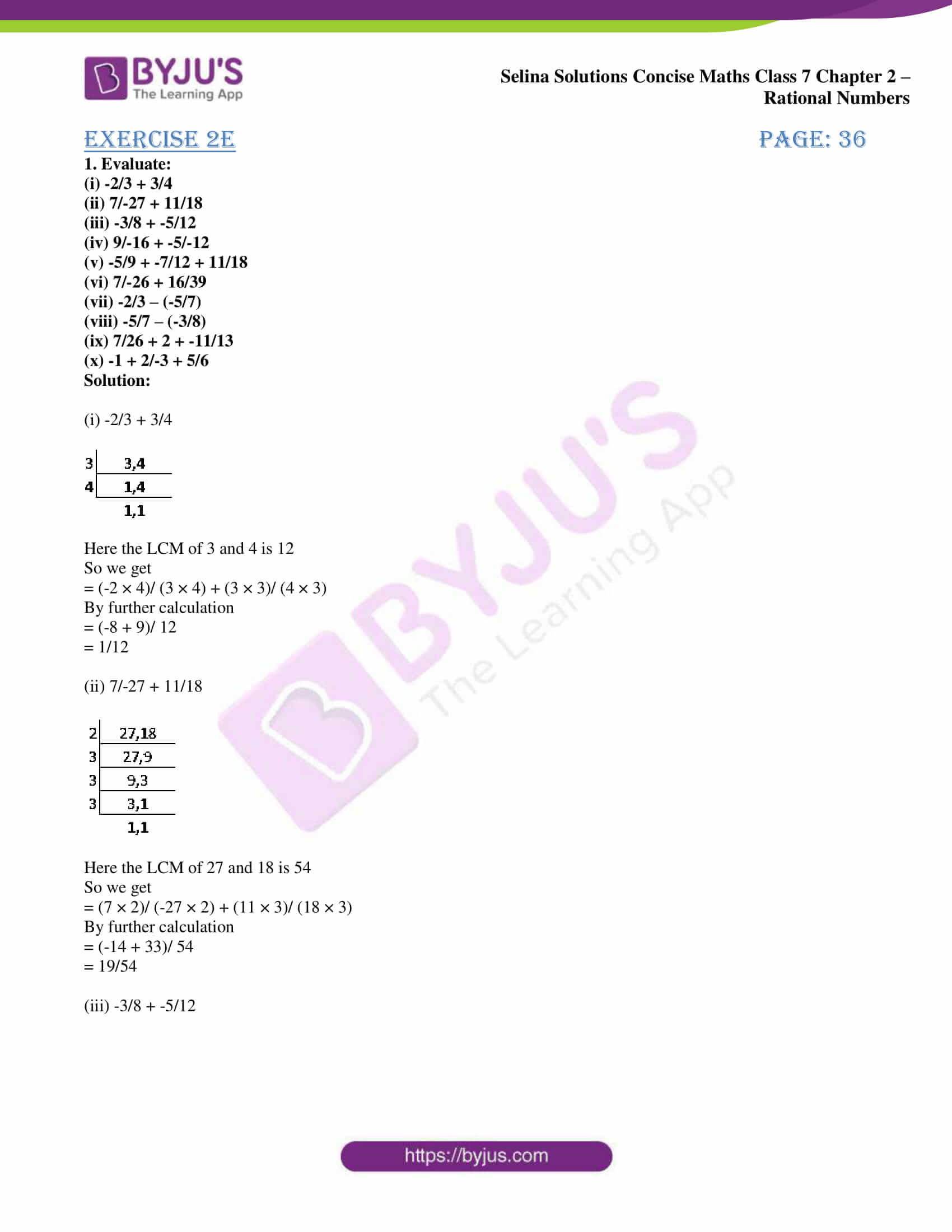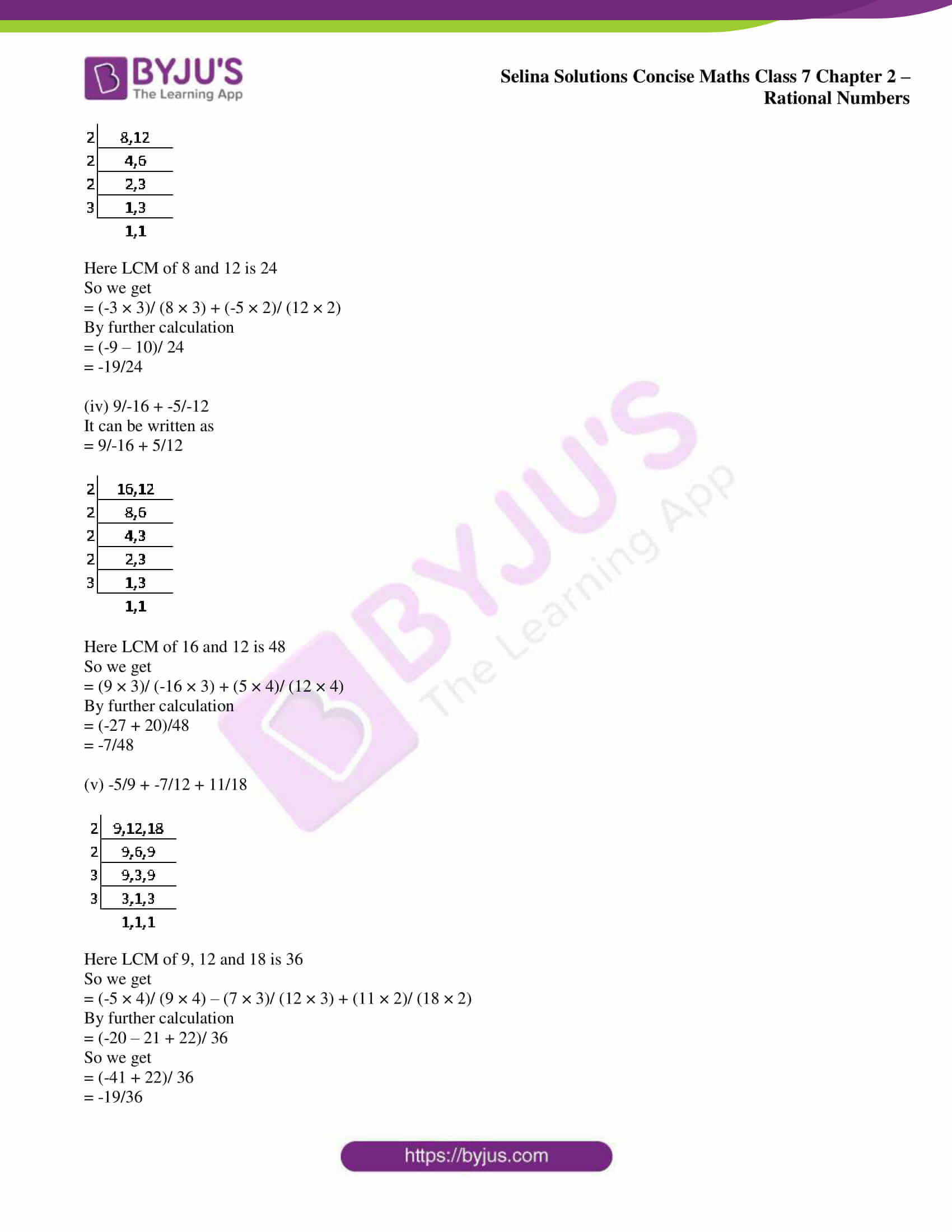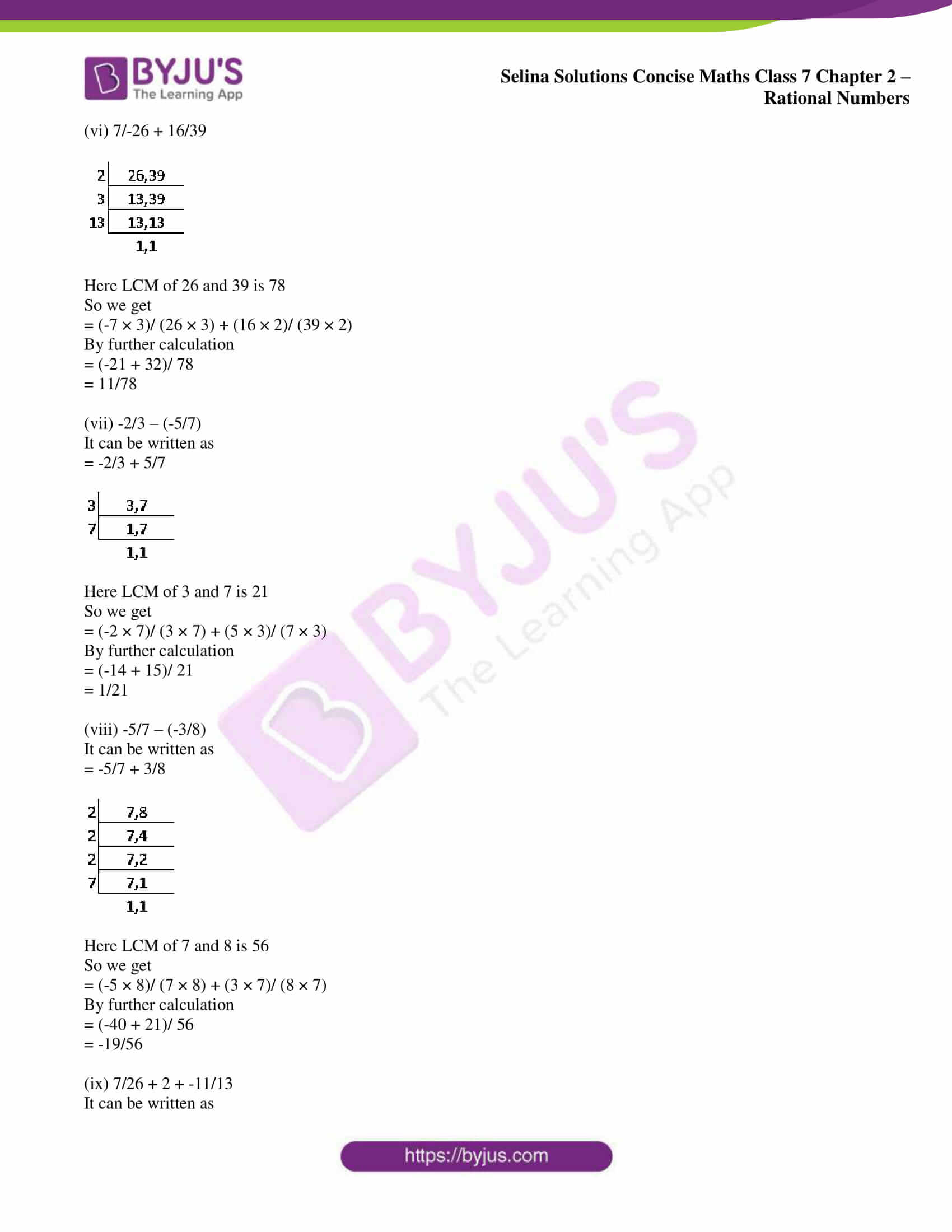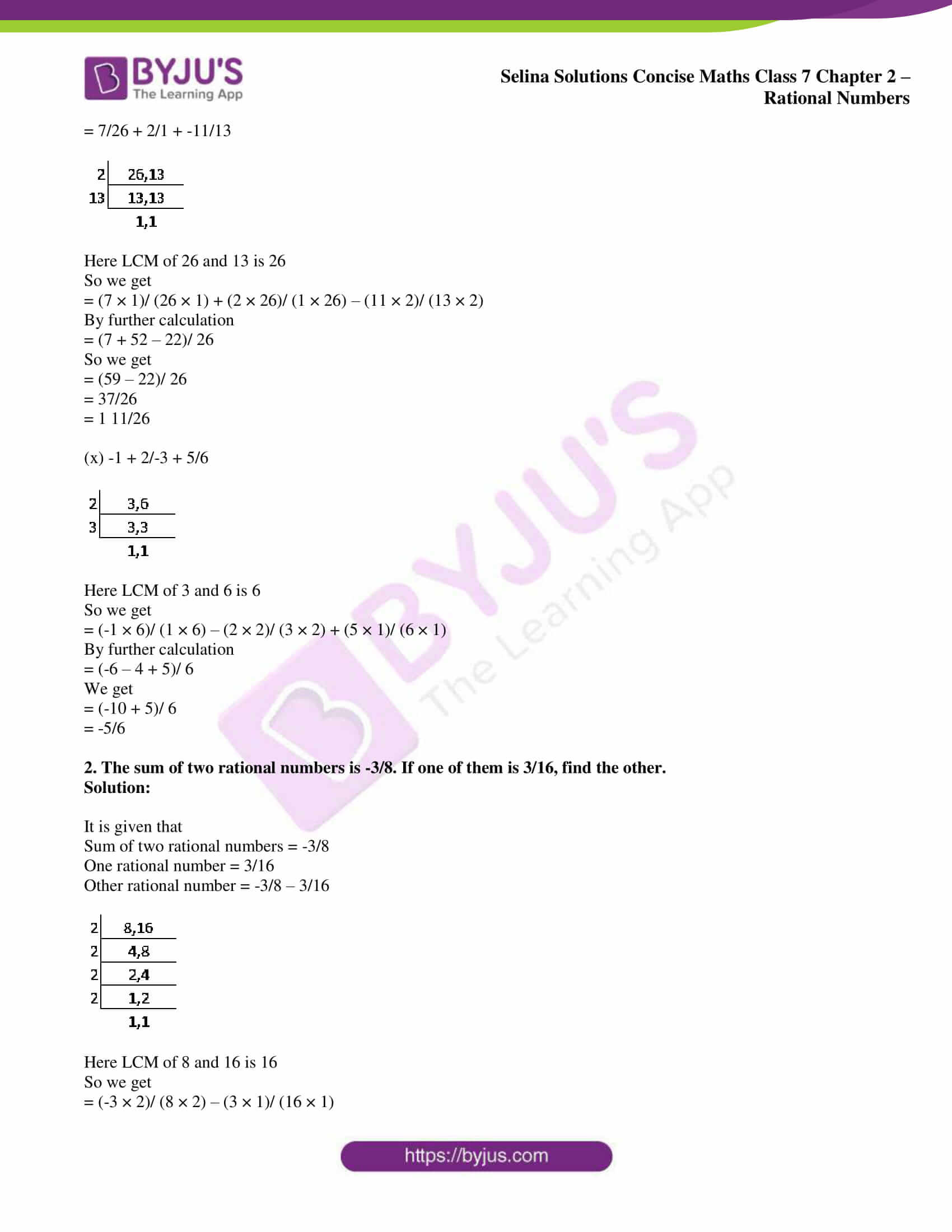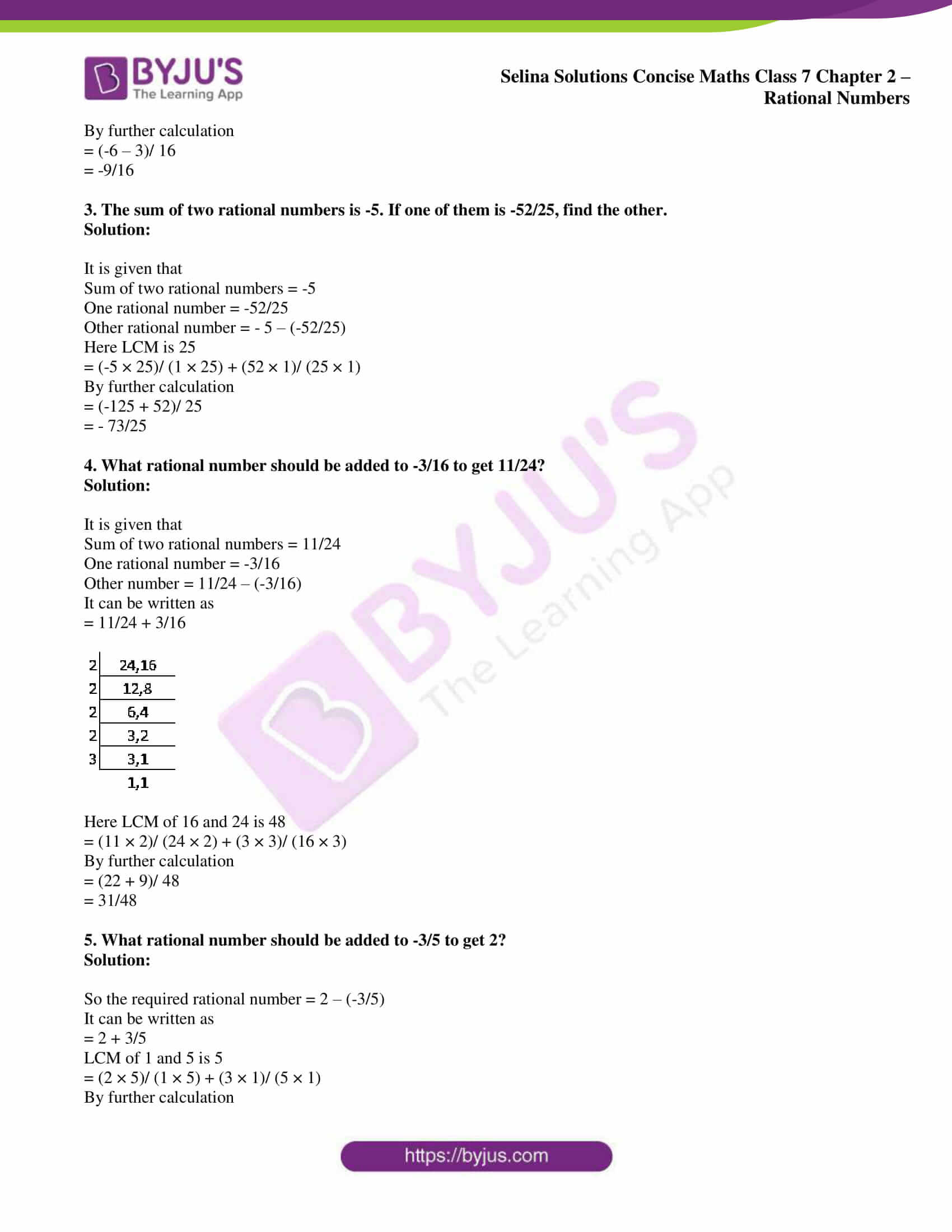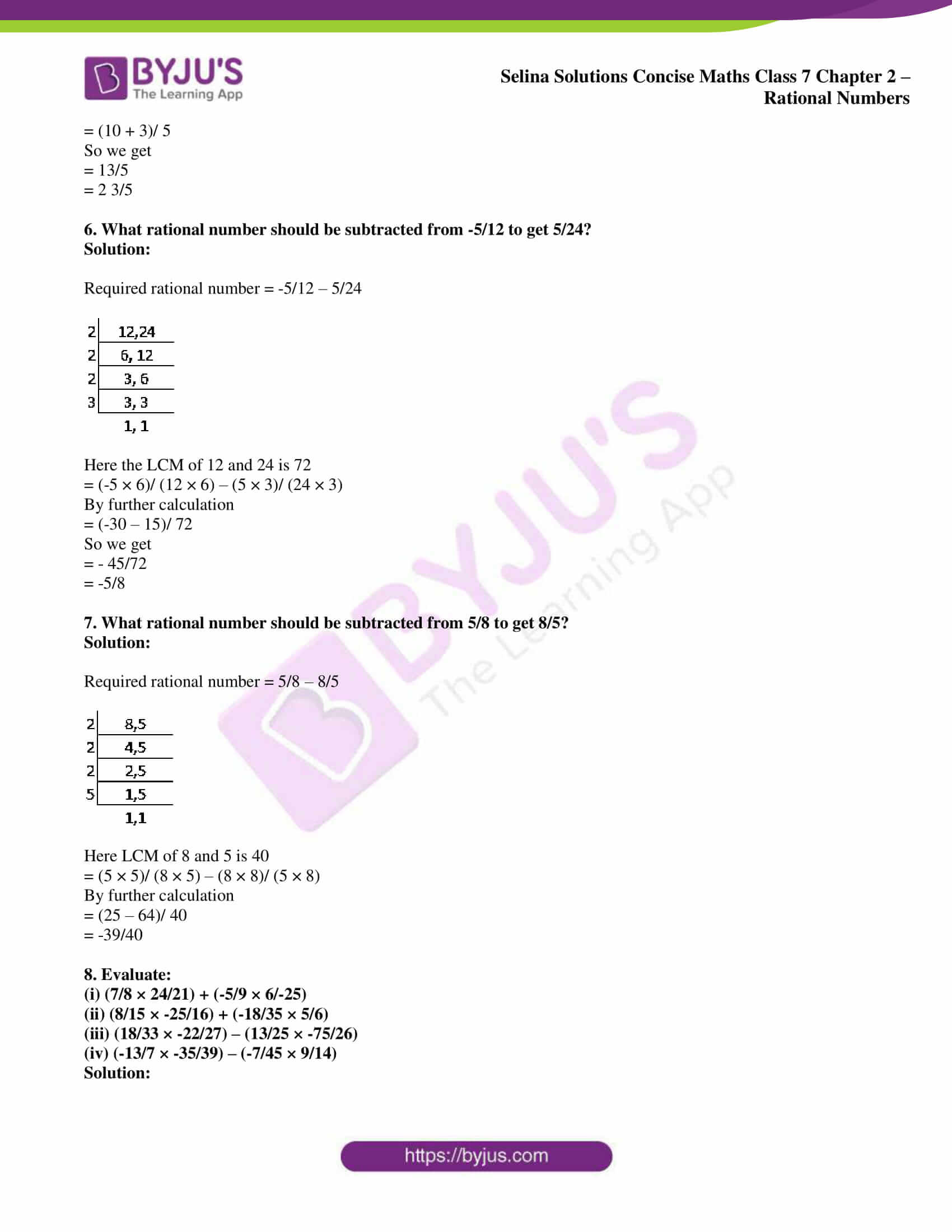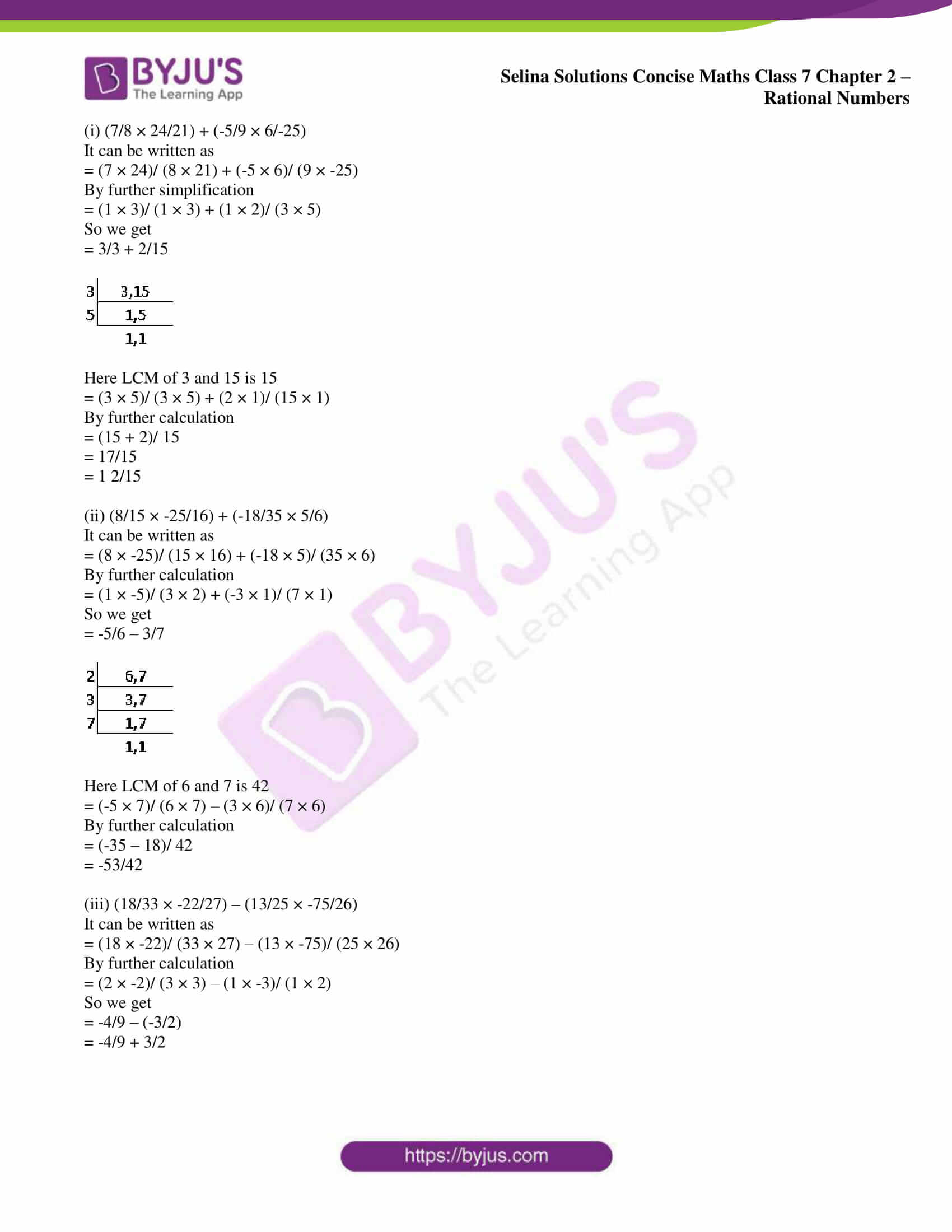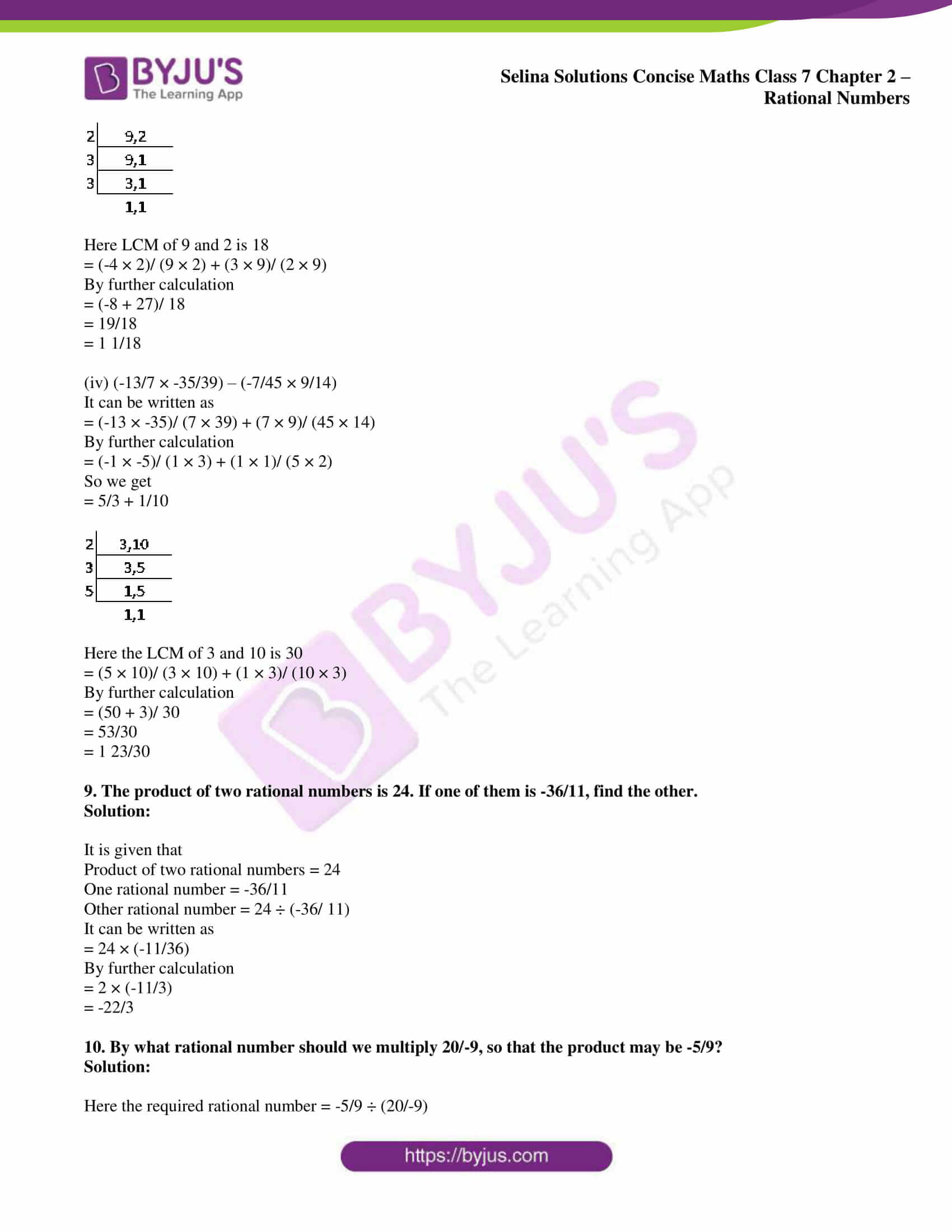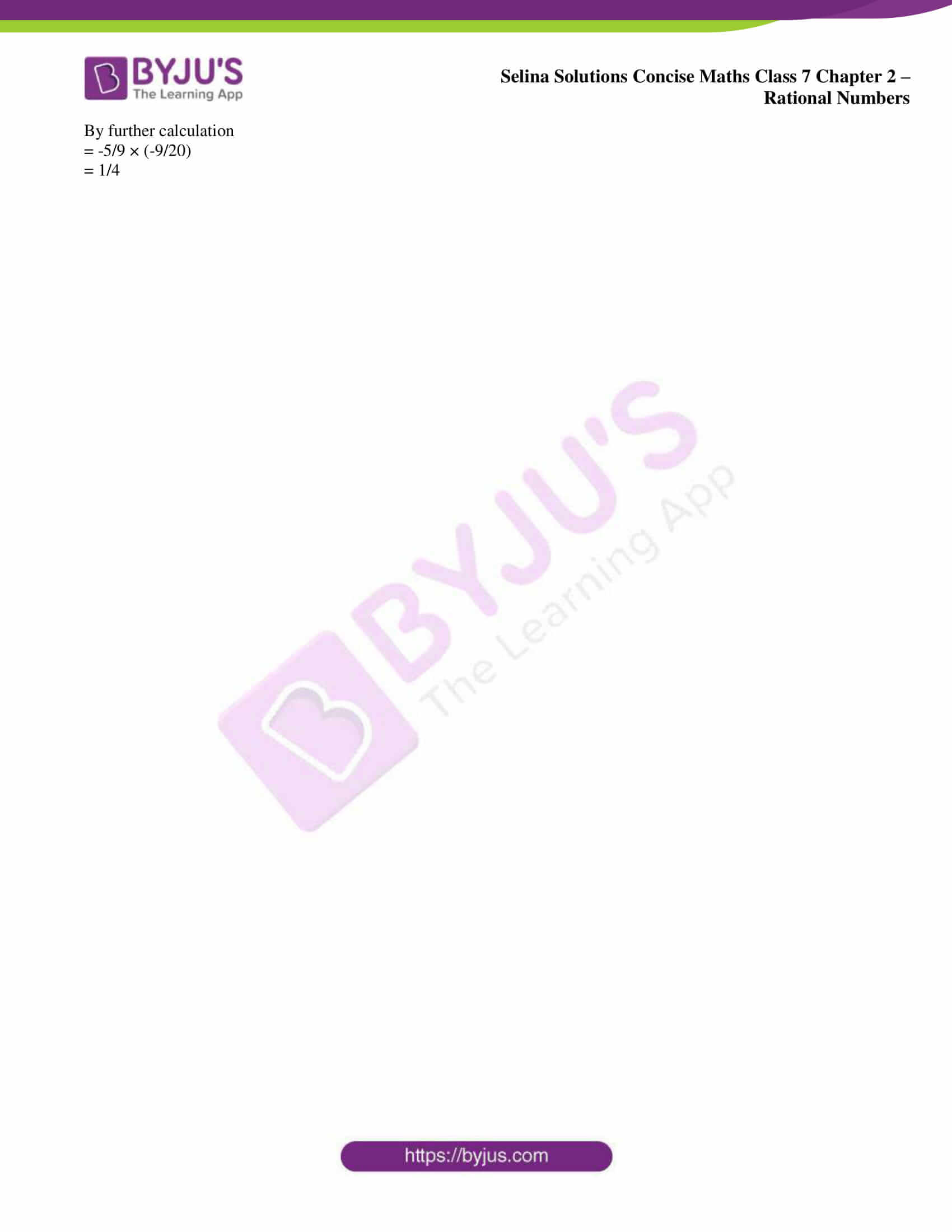### Access Selina Solutions Concise Maths Class 7 Chapter 2: Rational Numbers Exercise 2E

1. Evaluate:

(i) -2/3 + 3/4

(ii) 7/-27 + 11/18

(iii) -3/8 + -5/12

(iv) 9/-16 + -5/-12

(v) -5/9 + -7/12 + 11/18

(vi) 7/-26 + 16/39

(vii) -2/3 – (-5/7)

(viii) -5/7 – (-3/8)

(ix) 7/26 + 2 + -11/13

(x) -1 + 2/-3 + 5/6

Solution:

(i) -2/3 + 3/4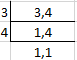Here the LCM of 3 and 4 is 12

So we get

= (-2 × 4)/ (3 × 4) + (3 × 3)/ (4 × 3)

By further calculation

= (-8 + 9)/ 12

= 1/12

(ii) 7/-27 + 11/18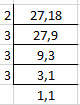Here the LCM of 27 and 18 is 54

So we get

= (7 × 2)/ (-27 × 2) + (11 × 3)/ (18 × 3)

By further calculation

= (-14 + 33)/ 54

= 19/54

(iii) -3/8 + -5/12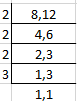Here LCM of 8 and 12 is 24

So we get

= (-3 × 3)/ (8 × 3) + (-5 × 2)/ (12 × 2)

By further calculation

= (-9 – 10)/ 24

= -19/24

(iv) 9/-16 + -5/-12

It can be written as

= 9/-16 + 5/12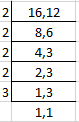Here LCM of 16 and 12 is 48

So we get

= (9 × 3)/ (-16 × 3) + (5 × 4)/ (12 × 4)

By further calculation

= (-27 + 20)/48

= -7/48

(v) -5/9 + -7/12 + 11/18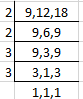Here LCM of 9, 12 and 18 is 36

So we get

= (-5 × 4)/ (9 × 4) – (7 × 3)/ (12 × 3) + (11 × 2)/ (18 × 2)

By further calculation

= (-20 – 21 + 22)/ 36

So we get

= (-41 + 22)/ 36

= -19/36

(vi) 7/-26 + 16/39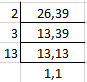Here LCM of 26 and 39 is 78

So we get

= (-7 × 3)/ (26 × 3) + (16 × 2)/ (39 × 2)

By further calculation

= (-21 + 32)/ 78

= 11/78

(vii) -2/3 – (-5/7)

It can be written as

= -2/3 + 5/7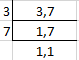Here LCM of 3 and 7 is 21

So we get

= (-2 × 7)/ (3 × 7) + (5 × 3)/ (7 × 3)

By further calculation

= (-14 + 15)/ 21

= 1/21

(viii) -5/7 – (-3/8)

It can be written as

= -5/7 + 3/8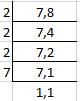Here LCM of 7 and 8 is 56

So we get

= (-5 × 8)/ (7 × 8) + (3 × 7)/ (8 × 7)

By further calculation

= (-40 + 21)/ 56

= -19/56

(ix) 7/26 + 2 + -11/13

It can be written as

= 7/26 + 2/1 + -11/13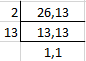Here LCM of 26 and 13 is 26

So we get

= (7 × 1)/ (26 × 1) + (2 × 26)/ (1 × 26) – (11 × 2)/ (13 × 2)

By further calculation

= (7 + 52 – 22)/ 26

So we get

= (59 – 22)/ 26

= 37/26

= 1 11/26

(x) -1 + 2/-3 + 5/6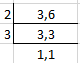Here LCM of 3 and 6 is 6

So we get

= (-1 × 6)/ (1 × 6) – (2 × 2)/ (3 × 2) + (5 × 1)/ (6 × 1)

By further calculation

= (-6 – 4 + 5)/ 6

We get

= (-10 + 5)/ 6

= -5/6

2. The sum of two rational numbers is -3/8. If one of them is 3/16, find the other.

Solution:

It is given that

Sum of two rational numbers = -3/8

One rational number = 3/16

Other rational number = -3/8 – 3/16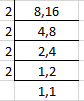Here LCM of 8 and 16 is 16

So we get

= (-3 × 2)/ (8 × 2) – (3 × 1)/ (16 × 1)

By further calculation

= (-6 – 3)/ 16

= -9/16

3. The sum of two rational numbers is -5. If one of them is -52/25, find the other.

Solution:

It is given that

Sum of two rational numbers = -5

One rational number = -52/25

Other rational number = – 5 – (-52/25)

Here LCM is 25

= (-5 × 25)/ (1 × 25) + (52 × 1)/ (25 × 1)

By further calculation

= (-125 + 52)/ 25

= – 73/25

4. What rational number should be added to -3/16 to get 11/24?

Solution:

It is given that

Sum of two rational numbers = 11/24

One rational number = -3/16

Other number = 11/24 – (-3/16)

It can be written as

= 11/24 + 3/16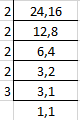Here LCM of 16 and 24 is 48

= (11 × 2)/ (24 × 2) + (3 × 3)/ (16 × 3)

By further calculation

= (22 + 9)/ 48

= 31/48

5. What rational number should be added to -3/5 to get 2?

Solution:

So the required rational number = 2 – (-3/5)

It can be written as

= 2 + 3/5

LCM of 1 and 5 is 5

= (2 × 5)/ (1 × 5) + (3 × 1)/ (5 × 1)

By further calculation

= (10 + 3)/ 5

So we get

= 13/5

= 2 3/5

6. What rational number should be subtracted from -5/12 to get 5/24?

Solution:

Required rational number = -5/12 – 5/24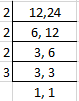Here the LCM of 12 and 24 is 72

= (-5 × 6)/ (12 × 6) – (5 × 3)/ (24 × 3)

By further calculation

= (-30 – 15)/ 72

So we get

= – 45/72

= -5/8

7. What rational number should be subtracted from 5/8 to get 8/5?

Solution:

Required rational number = 5/8 – 8/5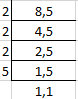Here LCM of 8 and 5 is 40

= (5 × 5)/ (8 × 5) – (8 × 8)/ (5 × 8)

By further calculation

= (25 – 64)/ 40

= -39/40

8. Evaluate:

(i) (7/8 × 24/21) + (-5/9 × 6/-25)

(ii) (8/15 × -25/16) + (-18/35 × 5/6)

(iii) (18/33 × -22/27) – (13/25 × -75/26)

(iv) (-13/7 × -35/39) – (-7/45 × 9/14)

Solution:

(i) (7/8 × 24/21) + (-5/9 × 6/-25)

It can be written as

= (7 × 24)/ (8 × 21) + (-5 × 6)/ (9 × -25)

By further simplification

= (1 × 3)/ (1 × 3) + (1 × 2)/ (3 × 5)

So we get

= 3/3 + 2/15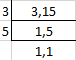Here LCM of 3 and 15 is 15

= (3 × 5)/ (3 × 5) + (2 × 1)/ (15 × 1)

By further calculation

= (15 + 2)/ 15

= 17/15

= 1 2/15

(ii) (8/15 × -25/16) + (-18/35 × 5/6)

It can be written as

= (8 × -25)/ (15 × 16) + (-18 × 5)/ (35 × 6)

By further calculation

= (1 × -5)/ (3 × 2) + (-3 × 1)/ (7 × 1)

So we get

= -5/6 – 3/7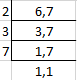Here LCM of 6 and 7 is 42

= (-5 × 7)/ (6 × 7) – (3 × 6)/ (7 × 6)

By further calculation

= (-35 – 18)/ 42

= -53/42

(iii) (18/33 × -22/27) – (13/25 × -75/26)

It can be written as

= (18 × -22)/ (33 × 27) – (13 × -75)/ (25 × 26)

By further calculation

= (2 × -2)/ (3 × 3) – (1 × -3)/ (1 × 2)

So we get

= -4/9 – (-3/2)

= -4/9 + 3/2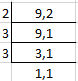Here LCM of 9 and 2 is 18

= (-4 × 2)/ (9 × 2) + (3 × 9)/ (2 × 9)

By further calculation

= (-8 + 27)/ 18

= 19/18

= 1 1/18

(iv) (-13/7 × -35/39) – (-7/45 × 9/14)

It can be written as

= (-13 × -35)/ (7 × 39) + (7 × 9)/ (45 × 14)

By further calculation

= (-1 × -5)/ (1 × 3) + (1 × 1)/ (5 × 2)

So we get

= 5/3 + 1/10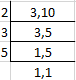Here the LCM of 3 and 10 is 30

= (5 × 10)/ (3 × 10) + (1 × 3)/ (10 × 3)

By further calculation

= (50 + 3)/ 30

= 53/30

= 1 23/30

9. The product of two rational numbers is 24. If one of them is -36/11, find the other.

Solution:

It is given that

Product of two rational numbers = 24

One rational number = -36/11

Other rational number = 24 ÷ (-36/ 11)

It can be written as

= 24 × (-11/36)

By further calculation

= 2 × (-11/3)

= -22/3

10. By what rational number should we multiply 20/-9, so that the product may be -5/9?

Solution:

Here the required rational number = -5/9 ÷ (20/-9)

By further calculation

= -5/9 × (-9/20)

= 1/4